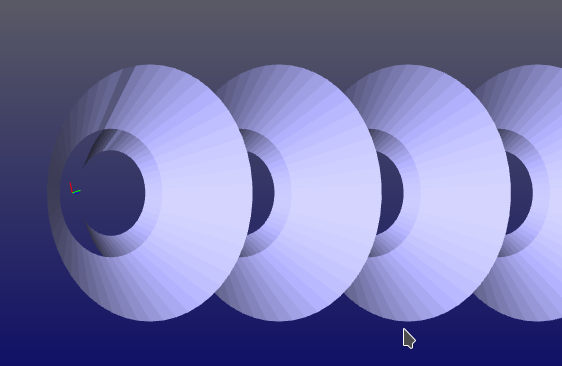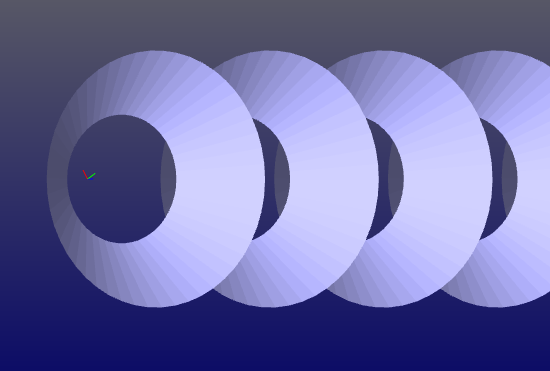# glWidget, strange behavior when drawing the first mesh in a loop with Lighting enabled

• Hello,

i have some strange behavior in my draw routines.
In several routines (different implementations) the first element that is illuminated like the normal is somehow wrong. (sometimes this also happens with lines, that do not have any normal at all)

Each function has a loop that draws some elements.
When i start the loop drawing not from 0 but from 1 for example the next second element is drawn wrong that looked fine when starting at zero.

Since it is is several functions / Implementations i'm not quite sure whats the problem.
Is this a known behavior? If not, if not i will prepare some minimal example that shows the effect.

These are the settings for my gl:

``````glEnable(GL_DEPTH_TEST);
glEnable(GL_BLEND);
glBlendFunc (GL_SRC_ALPHA, GL_ONE_MINUS_SRC_ALPHA);
glEnable(GL_LIGHTING);
glEnable(GL_LIGHT0);
glEnable(GL_NORMALIZE);
glEnable(GL_COLOR_MATERIAL);
glDisable(GL_CULL_FACE);``````

• Hi,

1. Qt version
2. OS name and version
3. Initialisation code

• Qt Version: Qt 5.5.1
Os: Ubuntu 16.04.2 LTS with KDE (Kubuntu)

``````void View3D::initializeGL()
{
qglClearColor(Qt::black);
glEnable(GL_DEPTH_TEST);
#ifdef TRANSPARENCY
glEnable(GL_BLEND); // Transparency
glBlendFunc (GL_SRC_ALPHA, GL_ONE_MINUS_SRC_ALPHA);
#endif
#ifdef LIGHTING
glEnable(GL_LIGHTING);
glEnable(GL_LIGHT0);
glEnable(GL_NORMALIZE);
glEnable(GL_COLOR_MATERIAL);
#endif
glDisable(GL_CULL_FACE);
}

void View3D::paintGL()
{
glClear(GL_COLOR_BUFFER_BIT | GL_DEPTH_BUFFER_BIT);
drawBackground();
glRotatef(90.0, 0.0, 1.0, 0.0);
// zoom
glScalef(zoom, zoom, zoom);
// x y z switched since rotated.
glTranslated(0, -yPos, +xPos);
// rotate
glRotatef(xRot, 1.0, 0.0, 0.0);
glRotatef(yRot, 0.0, 1.0, 0.0);
glRotatef(zRot, 0.0, 0.0, 1.0);
draw();
}``````

• Here is an small example how it looks like

``````drawCone(2.0);
drawCone(3.0); //drawCone see below
``````

note that the cones are drawn in a loop and it only happens to render strange with the first cone drawn in the loopIf i run the draw routine only once, it will not occure:

``````drawCone(3.0);
``````this is the function that draws the cones:

``````void View3D::drawCone(double x) const
{
glEnable(GL_LIGHTING);
// eigen3 Matrix (since all my data that i want to render comes from eigen calculations)
Vector4d p0;
Vector4d p1;
Vector4d p2;
Vector4d p3;
Vector4d color(0.7, 0.7, 1, 1.0);
uint nRays;
uint segments = 50;
// do some useless loop to show it only happens sometimes
for(int z=0;z<1000;z +=100)
{
// some function that generates a ring pattern
// row(0) = x, row(1) = y row(2) =z row(3) = ones
Matrix4Xd points_1 = ringPatternPos(&nRays, 10.0*x, 1, segments);
points_1.row(2) = VectorXd::Constant(1, nRays, 30.0+z);
Matrix4Xd points_2 = ringPatternPos(&nRays, 10.0*x, 1, segments);
// make cone from cylinder (with cylinders the strange behavior also doesn't occure !!
points_2.row(0) = points_2.row(0).array() * 2;
points_2.row(1) = points_2.row(1).array() * 2;
points_2.row(2) = VectorXd::Constant(1, nRays, 60.0+z);
// starts from 1 since first segment that is in ringpattern is the center point
for(uint seg = 1; seg<segments; seg++)
{
p0 = points_1.col(seg);
p1 = points_1.col(seg+1);
p2 = points_2.col(seg+1);
p3 = points_2.col(seg);
}
// close loop between last segment and first segment
p0 = points_1.col(segments);
p1 = points_1.col(1);
p2 = points_2.col(1);
p3 = points_2.col(segments);
}
}
``````

And the drawQuad() and setNormal() routines... nothing special here

``````inline void View3D::drawQuad(Vector4d p0, Vector4d p1, Vector4d p2, Vector4d p3, Vector4d color) const
{
glColor4f(color(0),color(1),color(2),color(3));
glVertex3f(p0(0), p0(1), p0(2));
glVertex3f(p1(0), p1(1), p1(2));
glVertex3f(p2(0), p2(1), p2(2));
glVertex3f(p3(0), p3(1), p3(2));
#ifdef LIGHTING
setNormal(p0, p1, p2);
#endif
glEnd();
}
``````
``````inline void View3D::setNormal(Vector4d p0, Vector4d p1, Vector4d p2) const
{
GLfloat n;
GLfloat a;
GLfloat b;
for(int i=0;i<4;i++)
{
a[i] = p1(i)-p0(i);
b[i] = p2(i)-p0(i);
}
n = a*b - a*b;
n = a*b - a*b;
n = a*b - a*b;
normalize3(n);
glNormal3f(n, n, n);
}
``````

• 5.5.1 is a bit outdated, can test against a more recent version of Qt ? 5.8.0 is the current version.

• I could solve the Problem.

I had the definiton of the normal at the wrong Position

``````glBegin(GL_TRIANGLES);
glColor4f(color(0),color(1),color(2),color(3));
glVertex3f(p2(0), p2(1), p2(2));
glVertex3f(p1(0), p1(1), p1(2));
glVertex3f(p0(0), p0(1), p0(2));
#ifdef LIGHTING
setNormal(p0, p2, p1);
#endif
glEnd();
``````

``````glBegin(GL_TRIANGLES);
#ifdef LIGHTING
setNormal(p0, p2, p1);
#endif
glColor4f(color(0),color(1),color(2),color(3));
glVertex3f(p2(0), p2(1), p2(2));
glVertex3f(p1(0), p1(1), p1(2));
glVertex3f(p0(0), p0(1), p0(2));
glEnd();
``````

now everything works fine

• Glad you found out and thanks for sharing !

Since you have it working now, please mark the thread as solved using the "Topic Tools" button so that other forum users may know a solution has been found :)